# Chapter 5 Factorisation of Algebraic Expressions RD Sharma Solutions Exercise 5.1 Class 9 Maths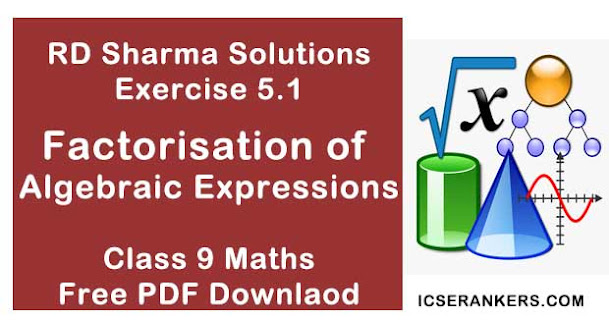Chapter Name RD Sharma Chapter 5 Factorisation of Algebraic Expressions Exercise 5.1 Book Name RD Sharma Mathematics for Class 10 Other Exercises Exercise 5.2Exercise 5.3Exercise 5.4 Related Study NCERT Solutions for Class 10 Maths

### Exercise 5.1 Solutions

Factorize :

1. x3 + x – 3x2 - 3

Solution

x3 + x – 3x2 - 3
Taking x common in (x3 + x)
= x(x2 + 1) - 3x2 - 3
Taking -3 common in (-3x2 - 3)
= x(x2 +1) - 3(x2 + 1)
Now, we take (x2 + 1) common
= (x2 + 1)(x - 3)
∴ x3 + x - 3x2 - 3 = (x2 + 1)(x - 3)

2. a(a + b)3 - 3a2 b(a +b)

Solution

Taking (a + b) common in two terms
= (a + b){a(a + b)2 - 3a2b}
Now, using (a + b)2 = a2 + b2 + 2ab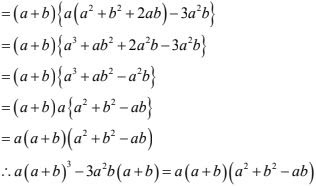3. x(x3 - y3 ) + 3xy(x - y)

Solution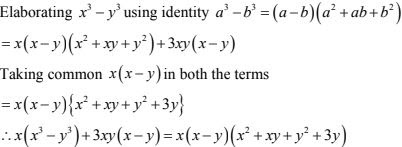4. a2x2 + (ax2 + 1)x + a
Solution
We multiply x(ax2 + 1) = ax3 + x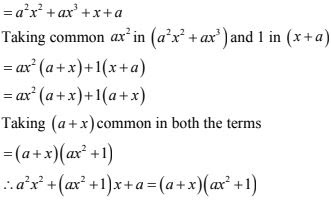5. x2 + y - xy - x
Solution
On rearranging
x2 + y - xy - x
Taking x common in the (x2 + y) and -1 in (-x + y)
= x( x - y)-1(x - y)
Taking (x - y) common in both the terms
= (x - y)(x - 1)
∴ x2 + y - xy - x = (x- y)(x - 1)

6. x3 - 2x2y + 3xy2 - 6y3
Solution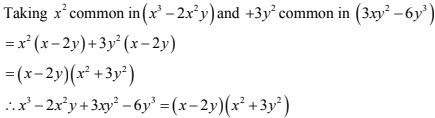7. 6ab - b2 + 12ac - 2bc
Solution
Taking common b in (6ab - b2 ) and 2c in (12ac - 2bc)
= b(6a - b) + 2c(6a - b)
Taking (6a - b) common in both terms
= (6a - b)(b + 2c)
∴ 6ab - b2 + 12ac - 2bc = (6a - b)(b + 2c)

8. [x2 + 1/x2 ] - 4[x + 1/x] + 6
Solution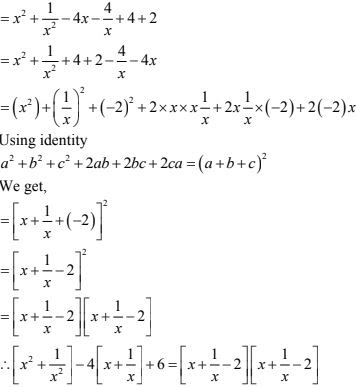9. x(x - 2)(x - 4) + 4x - 8
Solution
= x(x - 2)(x - 4) + 4(x - 2)
Taking (x - 2)common in both terms
= (x - 2){x(x - 4)+ 4}
= (x - 2){x2 - 4x + 4}
Now splitting middle term of x2 - 4x + 4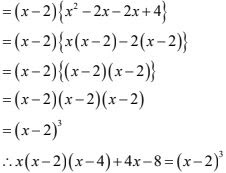10. (x + 2)(x2 + 25) - 10x2 - 20x
Solution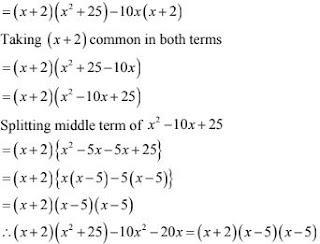11. 2a2 + 2√6ab + 3b2
Solution12. (a - b + c)2 + (b - c + a)2 + 2(a - b + c)(b - c + a)
Solution
Let (a - b + c) = x and (b - c + a) = y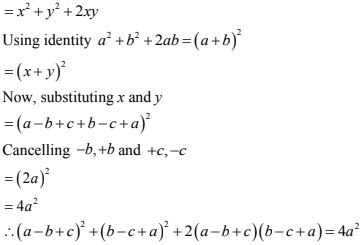13. a2 + b2 2(ab + bc + ca)
Solution14. 4(x - y)2 - 12(x - y)(x + y) + 9(x + y)2
Solution
Let (x - y) = a, (x + y) = b
= 4a2 - 12ab + 9b2
Splitting middle term -12 = -6 - 6
also 4× 9 = -6 × - 6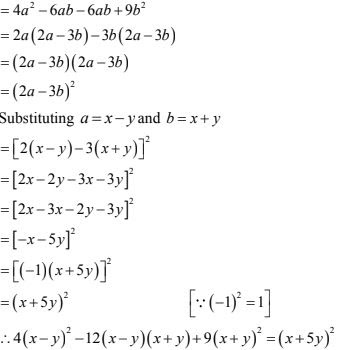15. a2 - b2 + 2bc - c2
Solution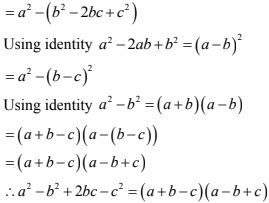16. a2 + 2ab + b2 - c2
Solution
Using identity a2 + 2ab + b2 = (a + b)2
= (a + b)2 - c2
Using identity a2 - b2 =(a +b)(a - b)
= (a + b + c)(a + b - c)
∴ a2 + 2ab + b2 - c2 = (a + b + c)(a + b -c)

17. a2 + 4b2 - 4ab - 4ac2
Solution
On rearranging
= a2 - 4ab + 4b2 - 4c2
= (a)2 - 2× a × 2b + (2b)2 - 4c2
Using identity a2 - 2ab + b2 = (a - b)2
= (a - 2b)2 - 4c2
= (a - 2b)2 - 2c2
Using identity a2 - b2 = (a + b)(a -b)
= (a - 2b + 2c)(a - 2b - 2c)
∴ a2 + 4b2 - 4ab - 4c2  = (a - 2b +2c)(a - 2b - 2c)

18. xy9 - yx9
Solution
xy9 - yx9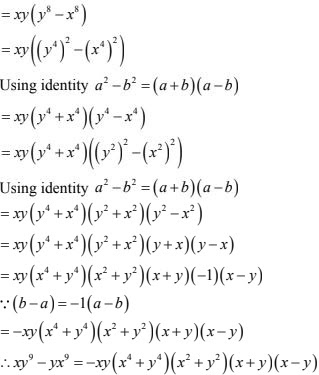19. x4 + x2y2 + y4
Solution
Adding x2 y2 and subtracting x2y2  to the given equation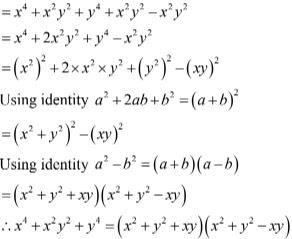20. x2 - y2 - 4xz + 4z2
Solution
On rearranging the terms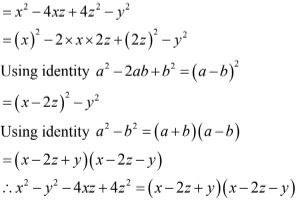21. x2 + 6√2 x + 10
Solution
Splitting middle term,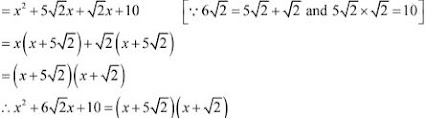22. x2 - 2√2x - 30
Solution
Splitting the middle term,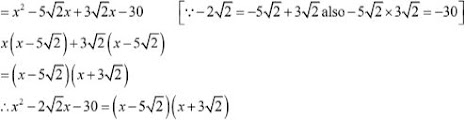23. x2  - √3x - 6
Solution
Splitting the middle term,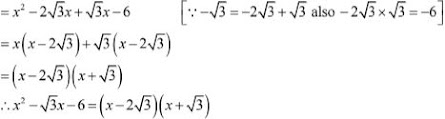24. x2  + 5√5x + 30
Solution
Splitting the middle term,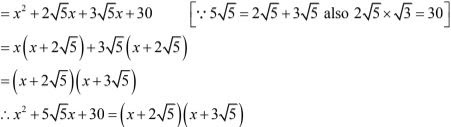25. x2  + 2√3x - 24
Solution
Splitting the middle term,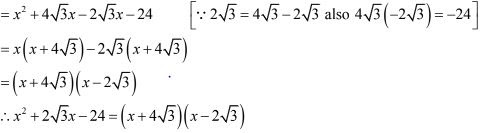26. 2x2  - 5/6 x + 1/12
Solution
Splitting the middle term,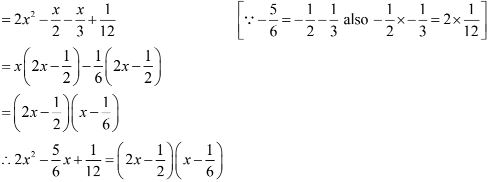27. x2  + 12/35 x + 1/35
Solution
Splitting the middle term,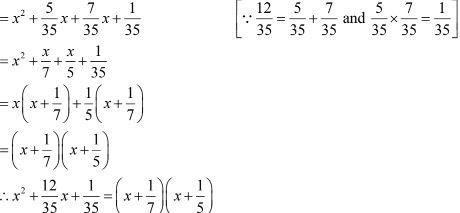28. 21x2  - 2x + 1/21
Solution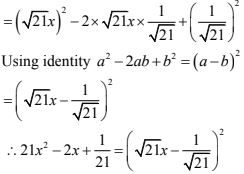29. 5√5x2  + 20x + 3√5
Solution
Splitting the middle term,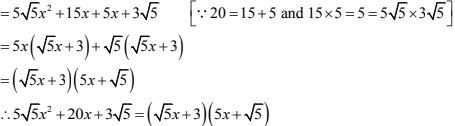30. 2x2 + 3√5x + 5
Solution
Splitting the middle term,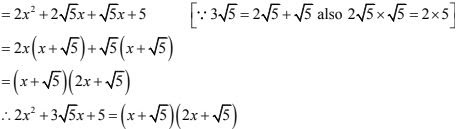31. 9(2a - b)2  - 4(2a - b) - 13
Solution
Let 2a - b = x
9x2  - 4x - 13
Splitting the middle term,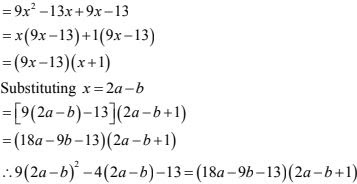32. 7(x - 2y)2 - 25(x - 2y) + 12
Solution
Let x - 2y = P
= 7P2 - 25P + 12
Splitting the middle term,
= 7P2 - 21P - 4P + 12
= 7P (P - 3) -4(P - 3)
= (P - 3)(7P - 4)
Substituting P = x - 2y
= (x - 2y - 3){7(x - 2y) - 4)
= (x - 2y - 3)(7x - 14y - 4)
∴ 7(x - 2y)2 - 25(x - 2y) + 12 = (x - 2y - 3)(7x - 14y - 4)

33. 2(x + y)2 - 9(x + y) - 5
Solution
Let x + y = z
= 2z2  - 9z - 5
Splitting the middle term,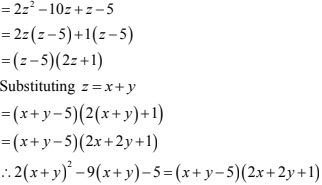34. Given possible expressions for the length and breadth of the rectangle having 35y2 + 13y - 12 as its area.
Solution
Area = 35y2  + 13y - 12
Splitting the middle term,
Area = 35y2 + 28y - 15y - 12
= 7y(5y + 4) - 3(5y + 4)
Area = (5y + 4)(7y - 3)
Also area of rectangle  = Length × Breadth
∴  Possible length = (5y + 4) and breadth = (7y - 3)
Or Possible length = (7y - 3) and breadth = (5y + 4)

35. What are the possible expressions for the dimensions of the cuboid whose volume is 3x2 - 12x.
Solution
Here volume = 3x2  - 12x
= 3x(x - 4)
= 3× x(x-4)
Also volume = Length × Breadth × Height
∴ Possible expressions for dimensions of the cuboid are = 3, x, (x - 4)